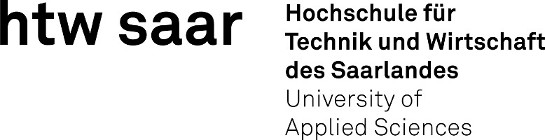Back to Main Page

Choose Module Version:

## The Statistics and Theory of Numerical Simulation

 Module name (EN): The Statistics and Theory of Numerical Simulation Degree programme: Engineering and Management, Master, ASPO 01.10.2019 Module code: MAM_19_A_1.01.MTS Hours per semester week / Teaching method: 5V+3U (8 hours per week) ECTS credits: 8 Semester: 1 Mandatory course: yes Language of instruction: German Assessment: Written exam 120 min. [updated 04.11.2020] Curricular relevance: DFMME-110 Mechanical Engineering, Master, ASPO 01.10.2019, semester 1, mandatory course MAM_19_A_1.01.MTS Engineering and Management, Master, ASPO 01.10.2019, semester 1, mandatory course Workload: 120 class hours (= 90 clock hours) over a 15-week period.The total student study time is 240 hours (equivalent to 8 ECTS credits).There are therefore 150 hours available for class preparation and follow-up work and exam preparation. Recommended prerequisites (modules): None. Recommended as prerequisite for: MAM_19_M_3.03.ASF Applied Numerical Simulations (Fluid Mechanics / Heat Transport)MAM_19_PE_2.04.SHY Hydraulic Servo-Motors [updated 10.03.2020] Module coordinator: Prof. Dr. Marco Günther Lecturer: Prof. Dr. Marco Günther [updated 21.03.2019] Learning outcomes: Statistics:After successfully completing this module, students will be able to solve statistical problems in the field of engineering sciences independently. They will be able to prepare and analyse complex data sets and interpret the results. Using suitable estimation methods, they will be able to draw conclusions about the population from a sample and critically scrutinize available statistics or the results of their evaluation.   Simulation TheoryIn the context of engineering problems, students will be familiar with the basics of mathematical modeling and numerical methods. They will be familiar with the basic properties of partial differential equations, simple solution methods and know about the possibilities and limitations of numerical methods using the finite difference method. [updated 04.11.2020] Module content: Statistics:- Descriptive statistics: central tendencies and dispersion, correlation, regression- Probability calculation: random variables und distributions, limit theorems- Inferential statistics: point estimate, interval estimate, testing hypotheses- Introduction to a statistics program package  Simulation Theory:- Fundamentals of vector analysis (repetition)- Fundamentals of partial differential equations (e.g. classification)- Basic concepts of numerics like stability, convergence, error- Solution methods: separable partial differential equation, Finite Differences Method (FDM)- Applying the FDM to boundary value problems and initial boundary value problems- Using Comsol Multiphysics as a solution tool [updated 04.11.2020] Teaching methods/Media: Statistics:Lecture: 3 hours per semester week, tutorials: 2 hours per semester week,  Use of the web-based learning software ActiveMath:http://markov.htw-saarland.de:8080/ActiveMath2/main/menu.cmd,  Simulation Theory:Lecture: 2 hours per semester week, Tutorials: 1 hour per semester week,  Blackboard, slides, handouts, tutorials [updated 04.11.2020] Recommended or required reading: Statistics:Weber H.: Einführung in die Wahrscheinlichkeit und Statistik für IngenieureHartung J., Elpelt B.: Multivariate StatistikWalz G., Grabowski B.: Lexikon der Stochastik mit BeispielenLecture notes _Deskriptive Statistik_, und Formelsammlung 1Lecture notes _Wahrscheinlichkeitsrechnung_ und Formelsammlung 2 Simulation Theory:Angermann A., Beuschel M, Rau M., Wohlfarth U.: MATLAB _ Simulink _ StateflowKnabner P., Angermann L.: Numerik partieller Differentialgleichungen [updated 04.11.2020]
```[Wed Apr 21 21:46:54 CEST 2021, CKEY=msutds, BKEY=mm2, CID=MAM_19_A_1.01.MTS, LANGUAGE=en, DATE=21.04.2021]
```